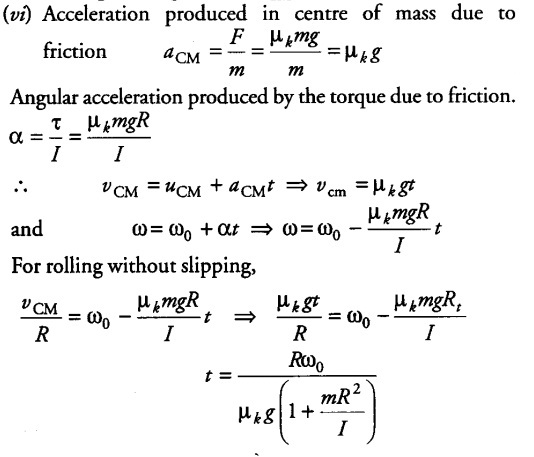# A disc of radius R is rotating with an angular speed to

A disc of radius R is rotating with an angular speed to, about a horizontal axis. It is placed on a horizontal table. The coefficient of kinetic friction is
(i) What was the velocity of its centre of mass before being brought in contact with the table?
(ii) What happens to the linear velocity of a point on its rim when placed in contact with the table?
(iii) What happens to the linear speed of the centre of mass when disc is placed in contact with the table?
(iv) Which force is responsible for the effects in (ii) and (iii)?
(v) What condition should be satisfied for rolling to begin?
(vi) Calculate the time taken for the rolling to begin.

(i) Before the disc is brought in contact with table, it is only rotating about its horizontal axis. So, its centre of mass is at rest i.e. vCM = 0.
(M) When rim is placed in contact with the table then its linear velocity at any point on the rim of disc will reduce due to kinetic friction.
{iii) If rotating disc is placed in contact with the table, its centre of mass acquires some velocity (which was zero before contact) due to kinetic friction. So linear velocity of CM will increase.
{iv) Kinetic friction.
{v) Rolling will begin when vCM = R(x).